Thread: Eric Dollard View Single Postt-rexSenior Member Join Date: Aug 2011 Location: In the bushes Posts: 422
Law of Electro-Magnetic Induction, Eight.

Magnetic Parameter Variation can be divided into two categories. One is the variation of the M.M.F., thru variation of the Current, i. The other is the variation of the Inductance thru variation of the Effective Path Permeability,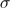. Current, i, and permeability factor,, are the two parameters which can undergo variation in order to give a variation in the quantity of Magnetism,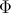.

The condition in which only the current undergoes variation exists with the Reactance Coil. Here the inductance is a constant, it is a factor of proportionality. This is expressed in the relation,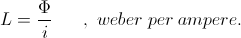Here a sine wave of current develops a sine wave of magnetism. Both waves exist in a direct proportion, L, and are thus in phase.

For the Reactance Coil the Law of Energy Conservation is satisfied. All the energy given to the magnetism is given back by the magnetism, no gain or loss. This movement of energy is facilitated by the induced E.M.F.

The condition in which only the Inductance undergoes the Parameter Variation exists with the Magnetic Amplifier, or the General Parametric Apparatus. Here the current is constant, it is a factor of proportionality. This is expressed by the relation,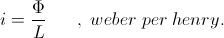Here a sine wave of Inductance Variation develops a sine wave of Magnetism. Both wave exist in direct proportion, i, and thus are in phase. Energy is exchanged thru the developed Induced E.M.F.

Here the Law of Energy Conservation is not satisfied. The Energy given to the Magnetism is not the Energy given back by the Magnetism, there is a gain or loos of Energy. In this situation the Law of Energy Continuity must be considered.

The condition in which the Magnetism is a constant is the Motor-Generator. This is expressed by the relation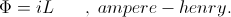Here it is both the Current, i, undergoing variation and the Inductance, L, undergoing variation, these in an Inverse Proportion in order to maintain a constant Magnetism. As sine wave of Inductance gives a sine wave of Current, but here the two waves are in Phase Opposition, that is, out of phase.

Because the Magnetism is Static no Energy is exchanged. Thus no Energy Law is involved in this condition of constant Magnetism. Hereby no E.M.F. is developed, the E.M.F. of the Motor-Generator is derived solely from the Rotation of the machine. The Law of Energy Continuity is involved here in that the Mechanical Energy consumed by the shaft re-appears as Electrical Energy produced by the armature windings, this representing a Generator. The reverse is true for a Motor. In each case the form of Energy is not conserved, it is consumed, or it is produced. Thus the Law of Energy Continuity expresses the Energy relationship.

For the condition of Inductance Parameter Variation and a constant Current the Magnetic Energy is not conserved. While for the Motor-Generator the Law of Energy Continuity is obvious, it is not so for the Parameter Variation apparatus. Here the Law of Energy Continuity is not identifiable, it is somewhat Indeterminate. This now brings into question the Law of Energy Perpetuity, this is to say Energy goes on forever just as it has existed forever As written in the Bible; As it was in the Beginning, so it shall be, for now and Ever-more:. The Law of Energy Perpetuity is similar to a Religious Law, to be defended and upheld by the Church. Amen.

BK DE N6KPH
__________________
SUPPORT ERIC DOLLARD'S WORK AT EPD LABORATORIES, INC.

Purchase Eric Dollard's Books & Videos - 70% of the sale goes to Eric and EPD Laboratories: Eric Dollard Books & Videos
Donate by Paypal: Donate to EPD Laboratories